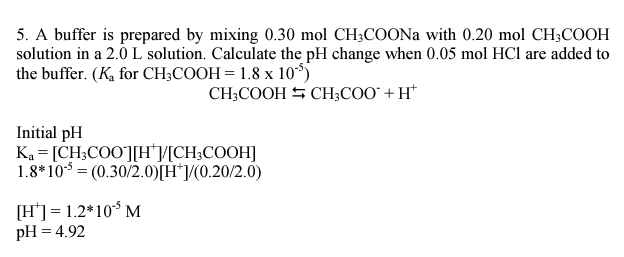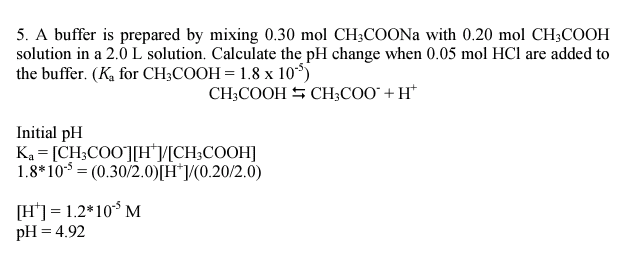# Buffer solution + equilibrium + Organic compound

For this question,why Ka =(ch3coo-)(h+)/(ch3cooh) instead of Ka =(ch3coo +x)(x)/(ch3cooh-x) ?Last edited by a moderator:

Borek
Mentor
why Ka =(ch3coo-)(h+)/(ch3cooh) instead of Ka =(ch3coo +x)(x)/(ch3cooh-x)

First equation uses equilibrium concentrations, second uses initial concentrations modified by the extent of the dissociation, to calculate equilibrium concentrations. Do you understand what these means?

epenguin
Homework Helper
Gold Member
For this question,why Ka =(ch3coo-)(h+)/(ch3cooh) instead of Ka =(ch3coo +x)(x)/(ch3cooh-x) ?You have now modified your initial post. I do not understand what you have in mind by your x.
You might have been confused by the CH3COONa. That is a conventional formula, it really just represents the composition of sodium acetate but in aqueous solution and for that matter in crystal sodium acetate is all Na+ and CH3COO-. When you add to this "HCl" - I.e. H+ + Cl- - a fraction of the acetate ions CH3COO- become protonated to form CH3COOH and then the calculations you are asked about involve that fraction, which actually determines the pH as you can see from the given formulae.

Last edited: